## DataRay Blog

September 15, 2016

# When to Use the D86 Beam Width Measurement Method

#### Introduction

Although the clip level method and the second moment method are the most popular methods of beam width measurement, other beam width measurement techniques such as the D86 method can be used with beam proﬁling cameras. Rather than using the 1D or 2D profile of the beam to determine the width, the D86 method uses the diameter of a 86.5% power enclosure as the beam width. In this blog post we describe the D86 beam width measurement method and the applications for which it is most appropriate.

#### Explanation of the D86 Method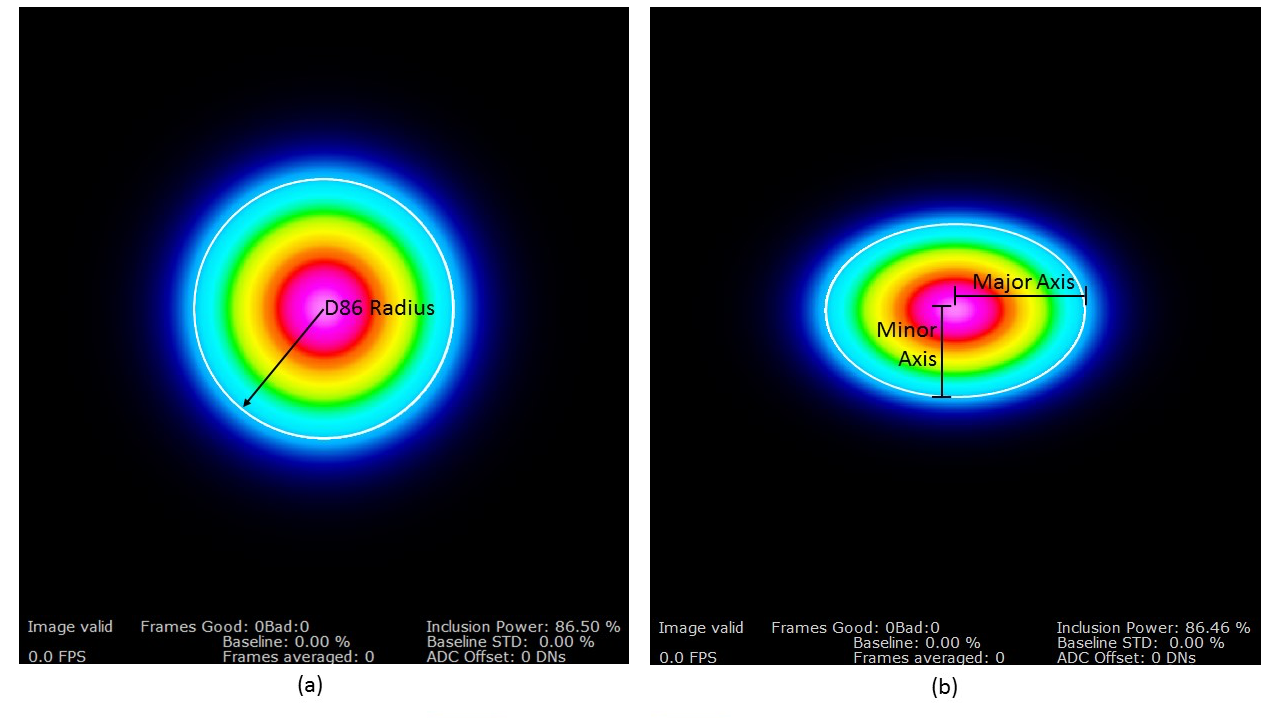Figure 1: (a) Radially symmetric TEM$$_{00}$$ Gaussian beam with the D86 beam enclosure (white line). The radius of the beam is given as the radius of the enclosure. (b) Elliptical TEM$${00}$$ Gaussian beam with the D86 enclosure (white line). The major and minor widths are given from the elliptical enclosure region’s major and minor axes.

The D86 method of beam width measurement uses a power enclosure, rather than the proﬁle of the beam to determine the beam width. To calculate the width of the beam with the D86 method, a circle (see Fig. 1a) or ellipse (see Fig. 1b) is first found which encloses 86.5% of the total power across the sensor. The D86 method is named after this percentage enclosure. Once the power enclosure has been found, the radius of the enclosure is used as the beam radius (in the case of the ellipse, the major and minor axes are used as the beam radius).

Although the choice of included power can seem arbitrary, the 86.5% value is related to the fundamental TEM 00 Gaussian beam’s 1/e 2 value (13.5%). Other beam measurement methods utilize the 1/e 2 value as well. The clip level method (often used with scanning slit devices) finds the two points where the beam intercepts 13.5% of the beam’s peak power and the difference between these two points is then used as the width. The second moment method—which is the most robust measurement method—calculates the beam width $$w(z)$$ with the equation

\begin{equation} w^2(z)=\frac{\displaystyle \iint r^2I(r, z)r \,dr\,d\theta}{\displaystyle \iint I(r, z)r \,dr\,d\theta}\text{,} \end{equation}

where $$I(r, z)$$ is the beam's intensity distribution. For a fundamental Gaussian beam, the width measured with the second moment yields the 1/$$e^2$$ value. Similarly, the D86 method returns the 1$$/e^2$$ value for a fundamental Gaussian beam. Even though the various width determination methods return similar values for the fundamental Gaussian, their values diverge for higher-order beams. We generated two beams, a TEM$$_{00}$$ Gaussian (see Fig. 2), and a TEM$$_{20}$$ Laguerre-Gaussian (see Fig. 3), and used DataRay's software to measure the diameters of the two beams with both the D86 method and the second moment method (see Table 1). The results of the test showed that, as predicted, the two beam width measurement methods return the same value for a fundamental Gaussian, but return significantly different values for the higher-order beams.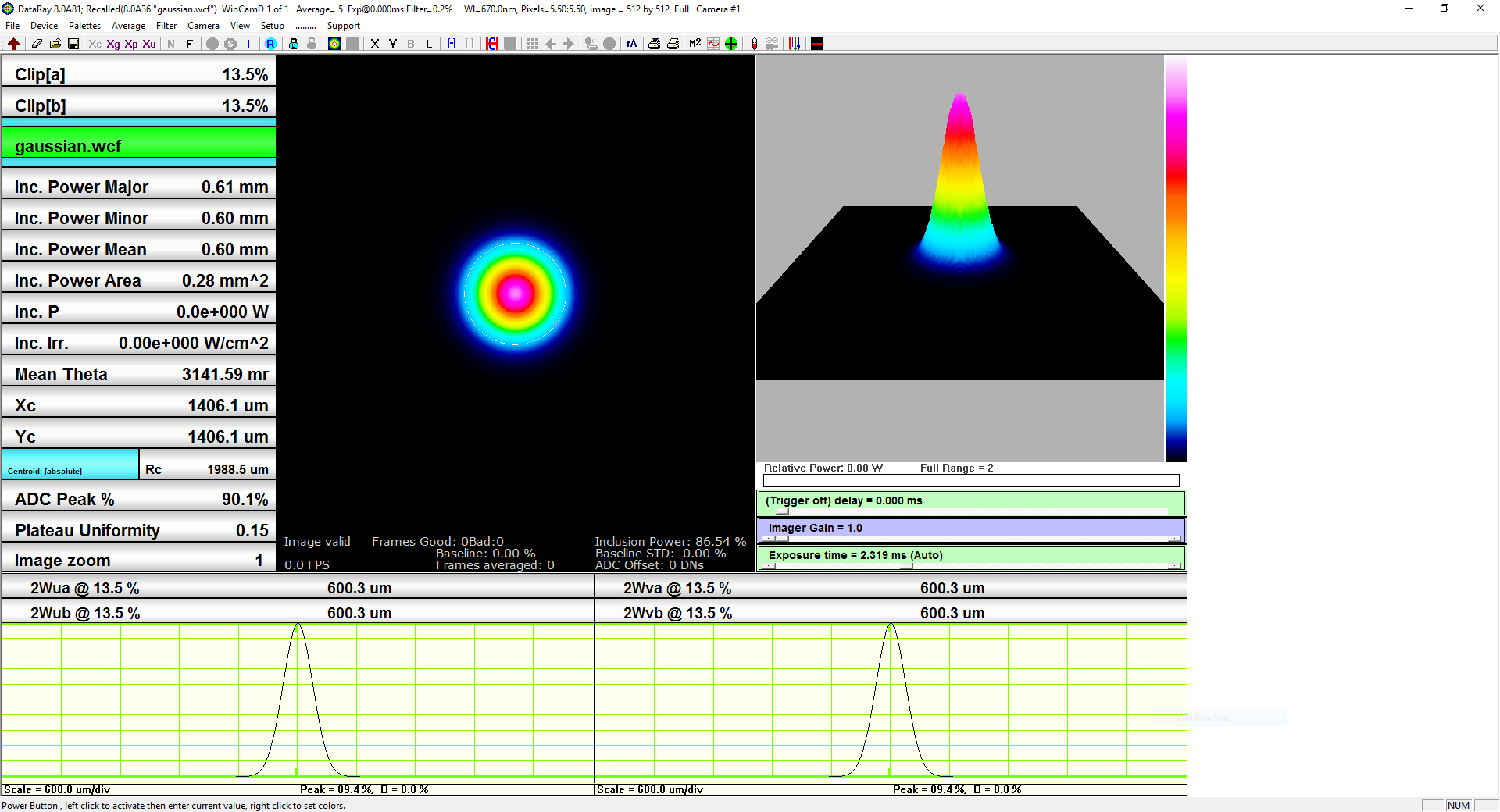Figure 2: DataRay software measuring the width of a fundamental TEM$$_{00}$$ Gaussian beam with the second moment method.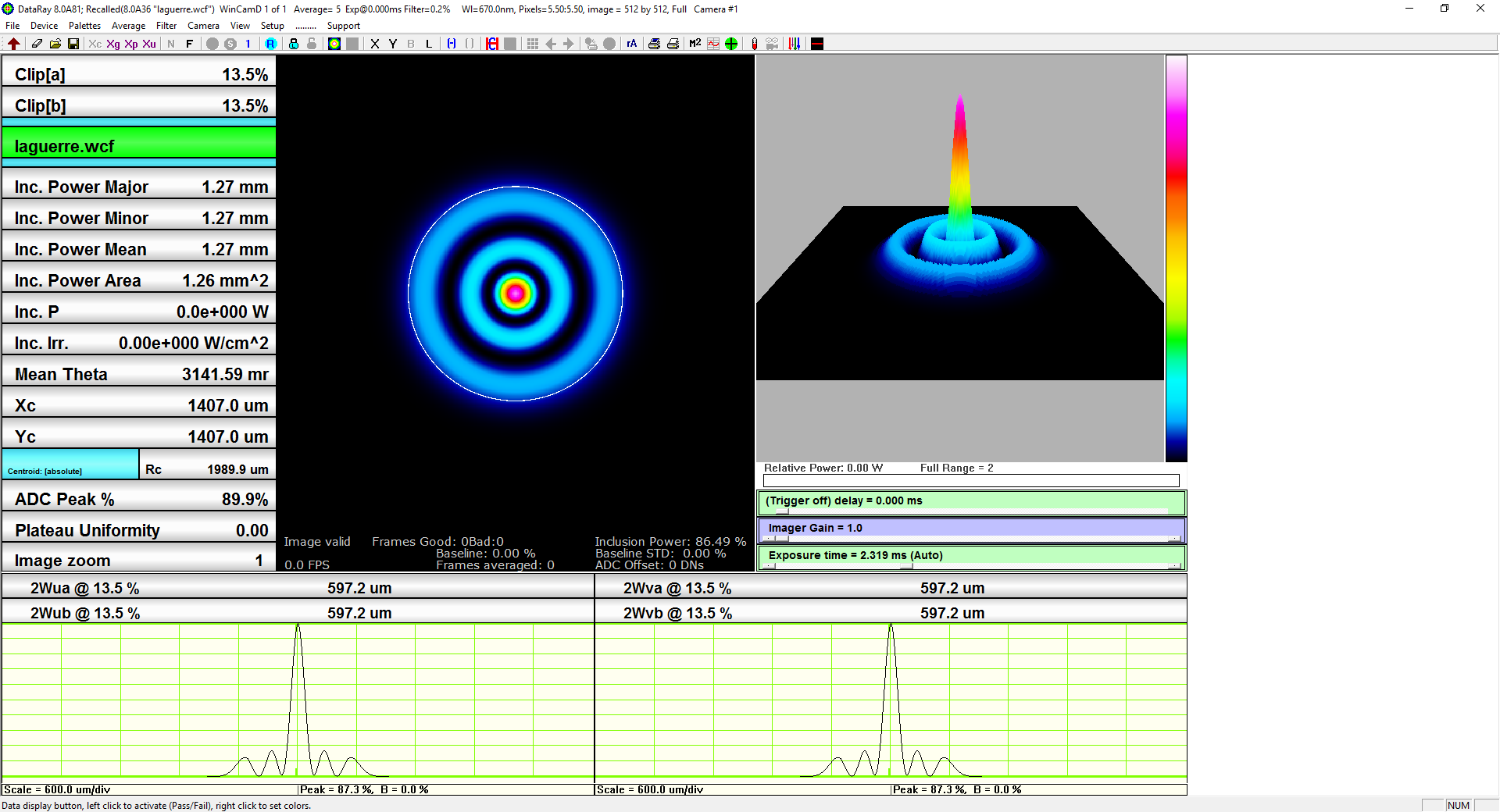Figure 3: DataRay software measuring the width of a TEM$$_{20}$$ Laguerre-Gaussian beam with the D86 method.
Second Moment D86
TEM$$_{00}$$ Gaussian 599 µm 600 µm
TEM$$_{20}$$ Laguerre-Gaussian 1358 µm 1270 µm
Table 1: Results of measuring the widths of the two simulated beams with both the second moment method and the D86 method.

#### D86 and M²

The ISO 11146 standard calls for using the second moment width when calculating M². If the D86 method is used instead, the resulting M² value will be inaccurate. The M² values for the TEM$$_{00}$$ Gaussian beam and the TEM$$_{20}$$ Laguerre-Gaussian beam were found using both the second moment method and the D86 method (see Table 2). Additionally, the theoretical M² values were calculated to compare the measured M² values against. The D86 method returned comparable results to the second moment method for the fundamental Gaussian beam, but its accuracy for higher-order beams was significantly diminished. This is particularly problematic since the M² value given by the D86 method is closer to 1 than the actual value, falsely indicating higher beam quality. For this reason, DataRay software offers the D86 method to measure the width of a beam, but does not include an option to measure M² with the D86 method.

Theoretical Second Moment D86
TEM$$_{00}$$ Gaussian 1 0.996 0.998
TEM$$_{20}$$ Laguerre-Gaussian 5 5.08 4.46
Table 2: Results of measuring the M² value for two simulated beams with both the second moment method and the D86 method.

#### Using D86 Mode in DataRay Software

To use the D86 method with DataRay software, first click Setup on the main menu. From the Setup menu select DXX mode (see Fig. 4a). The buttons on the main screen should be changed to reflect the new mode (see Fig. 3). Next, set the included power percent by selecting Set Included Power Percentage Target from the Setup menu. A dialog box should appear which allows the user to enter the included power target in percent (see Fig. 4b).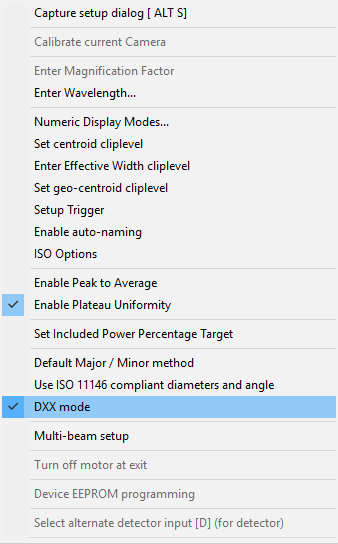(a)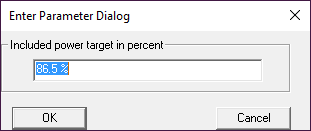(b)
Figure 4: (a) The menu that appears after selecting Setup from the DataRay software’s main screen. (b) The dialog box that appears after selecting Set Included Power Percentage Target from the Setup Menu.

#### Conclusion

While the D86 method is useful for certain applications, it should not be used as a replacement for the second moment method, due to the difference in beam width measurement values for higher-order beams. If you have any questions regarding beam measurement methods for your application please contact us. We have years of experience in laser beam proﬁling and would love to discuss a solution for your system.

Posted by: Lucas Hofer